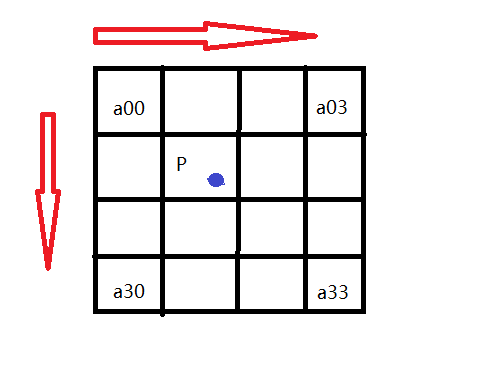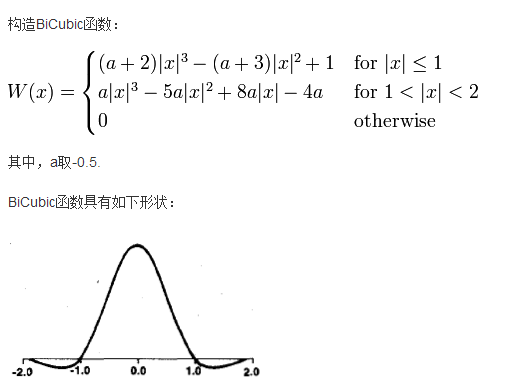# 图像缩放之双三次插值法

## 数学原理P的坐标为P(x+u,y+v)，其中x,y分别表示整数部分，u,v分别表示小数部分。那么我们就可以得到如图所示的k_i_0=W(1+u), k_i_1=W(u), k__i_2=W(1-u), k_i_3=W(2-u);
k_j_0=W(1+v), k_j_1=W(v), k_j_2=W(1-v), k_j_3=W(2-v);

k_i_j=k_i*k_j我们就可以得到每个像素a(i,j)对应的权值了。

pixelB(X,Y)=pixelA(0,0)*k_0_0+pixelA(0,1)*k_0_1+…+pixelA(3,3)*k_3_3;

## 程序实现

/**********************10-9*******************************

**********************************************************/

#include <opencv2\opencv.hpp>
#include <iostream>
#include <math.h>
using namespace std;
using namespace cv;

float a = -0.5;//BiCubic基函数
void getW_x(float w_x, float x);
void getW_y(float w_y, float y);

int main(){

float Row_B = image.rows*2;
float Col_B = image.cols*2;

Mat biggerImage(Row_B, Col_B, CV_8UC3);

for (int i = 2; i < Row_B-4; i++){
for (int j = 2; j < Col_B-4; j++){
float x = i*(image.rows / Row_B);//放大后的图像的像素位置相对于源图像的位置
float y = j*(image.cols / Col_B);

/*if (int(x) > 0 && int(x) < image.rows - 2 && int(y)>0 && int(y) < image.cols - 2){*/
float w_x, w_y;//行列方向的加权系数
getW_x(w_x, x);
getW_y(w_y, y);

Vec3f temp = { 0, 0, 0 };
for (int s = 0; s <= 3; s++){
for (int t = 0; t <= 3; t++){
temp = temp + (Vec3f)(image.at<Vec3b>(int(x) + s - 1, int(y) + t - 1))*w_x[s] * w_y[t];
}
}

biggerImage.at<Vec3b>(i, j) = (Vec3b)temp;
}
}

imshow("image", image);
imshow("biggerImage", biggerImage);
waitKey(0);

return 0;
}
/*计算系数*/
void getW_x(float w_x,float x){
int X = (int)x;//取整数部分
float stemp_x;
stemp_x = 1 + (x - X);
stemp_x = x - X;
stemp_x = 1 - (x - X);
stemp_x = 2 - (x - X);

w_x = a*abs(stemp_x * stemp_x * stemp_x) - 5 * a*stemp_x * stemp_x + 8 * a*abs(stemp_x) - 4 * a;
w_x = (a + 2)*abs(stemp_x * stemp_x * stemp_x) - (a + 3)*stemp_x * stemp_x + 1;
w_x = (a + 2)*abs(stemp_x * stemp_x * stemp_x) - (a + 3)*stemp_x * stemp_x + 1;
w_x = a*abs(stemp_x * stemp_x * stemp_x) - 5 * a*stemp_x * stemp_x + 8 * a*abs(stemp_x) - 4 * a;
}
void getW_y(float w_y, float y){
int Y = (int)y;
float stemp_y;
stemp_y = 1.0 + (y - Y);
stemp_y = y - Y;
stemp_y = 1 - (y - Y);
stemp_y = 2 - (y - Y);

w_y = a*abs(stemp_y * stemp_y * stemp_y) - 5 * a*stemp_y * stemp_y + 8 * a*abs(stemp_y) - 4 * a;
w_y = (a + 2)*abs(stemp_y * stemp_y * stemp_y) - (a + 3)*stemp_y * stemp_y + 1;
w_y = (a + 2)*abs(stemp_y * stemp_y * stemp_y) - (a + 3)*stemp_y * stemp_y + 1;
w_y = a*abs(stemp_y * stemp_y * stemp_y) - 5 * a*stemp_y * stemp_y + 8 * a*abs(stemp_y) - 4 * a;
}

## 效果展示12-282万+
11-1206-051万+
04-261458
01-252万+
08-20407
04-251万+
04-091594